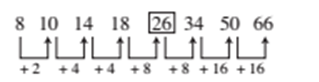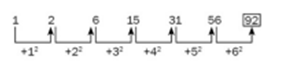# RRB ALP 2018 Practice Test Papers | Arithmetic Questions (Day-2)

Dear Aspirants, Here we have given the Important RRB ALP & Technicians Exam 2018 Practice Test Papers. Candidates those who are preparing for RRB ALP 2018 can practice these Arithmetic Questions to get more confidence to Crack RRB 2018 Examination.

[WpProQuiz 1441]

### Click “Start Quiz” to attend these Questions and view Explanation

1. If (a + 1/a)2 = 3, then the value of a3 + 1/a3 is—

(A) 0

(B) 9

(C) 3√3

(D) √3

1. 8, 10, 14, 18, (……), 34, 50, 66

(A) 24

(B) 25

(C) 26

(D) 27

1. If 40% of 3/4 of 4/5 of some number is 48, what would be the value of 1 per cent of that number?

(A) 1

(B) 2

(C) 10

(D) 20

1. What would be the value of log 9, if log 27 = 1·431?

(A) 0·934

(B) 0·945

(C) 0·954

(D) 0·958

1. The sum of first 8 terms of a geometric progression is 6560 and common ratio is 3. The first term will be—

(A) 1

(B) 2

(C) 3

(D) 4

1. A cricketer has some average of runs till 16 innings. He scored 85 runs in his 17th innings. Then his average increased by 3 runs. What is average of runs after 17th innings?

(A) 42

(B) 46

(C) 34

(D) 37

1. What is the sum of all the internal angles of a polygon with ‘8’ sides?

(A) 360o

(B) 180o

(C) 720o

(D) 1080o

1. 1, 2, 6, 15, 31, 56,?

(A) 65

(B) 85

(C) 90

(D) 92

1. Average age of P and Q is 42 years and average age of R and S is 52 years. What is the average of ages of P, Q, R and S?

(A) 49 years

(B) 53 years

(C) 51 years

(D) 47 years

1. If p is even and q is odd, then which of the following will necessarily be odd?

(A) p × q

(B) p + 2q

(C) p + q

(D) 2p + 2q

Explanation:

(a + 1/a)=√3

(a + 1/a)3 = a3 + 1/a3 + 3(a + 1/a)

=3√3-3√3=0

Explanation:Explanation:

40/100*3/4*4/5*x=48

X=200

1 % of x= 2

Explanation:

log 27 = 1og 33

3·log 3 = 1·431

⇒ log 3 = 1·431/3

∴ log 9 = log 32 = 2 log 3 = 2 × 1·431/3 = 2·862/3 = 0·954

Explanation:

First term of geometric progression = a

Sum of eight terms G. P. = a (38 – 1) / (3 – 1) = 6560

∴ a = 2 × 6560/ (38 – 1) = 2 × 6560 / (6561 – 1) = 2

Explanation:

Rise in average after 17th innings = 3 × Total increase in runs after 17th innings = 3 × 17 = 51

The player scored 85 runs in 17th innings.

∴ His average of runs till 16th innings = 85 – 51 = 34

∴ Average of all 17 innings = 34 + 3 = 37

Explanation:

Sum of all interior angles of a polygon of ‘n’ sides is (n–2) × 180o.

So, for ‘8’ sides, it is 6 × 180o = 1080o.

Explanation:Explanation:

Sum of ages of P and Q = 42 × 2 = 84 years.

Sum of ages of R and S = 52 × 2 = 104 years.

So, average of ages of P, Q, R and S = (84+104)/4=47

Explanation:

p × q = even × odd = even

p + 2q = even + 2 × odd (even) = even

p + q = even + odd = odd

2p + 2q = 2 × even (even) + 2 × odd (even) = even

RRB ALP 2018 Practice Test Papers | Arithmetic Questions (Day-1)